# Box-Cox Transformation and Target Variable: Explained – Built InBox-Cox transformation is a statistical technique that transforms your target variable so that your data closely resembles a normal distribution.

In many statistical techniques, we assume that the errors are normally distributed. This assumption allows us to construct confidence intervals and conduct hypothesis tests. By transforming your target variable, we can hopefully normalize our errors, if they are not already normal.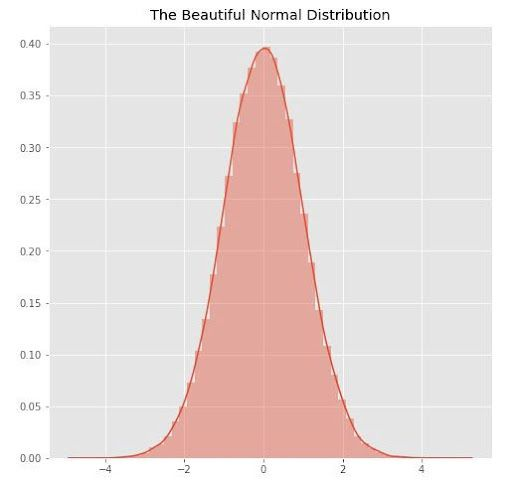An example of a normal distribution statistical graph. | Image: Andrew Plummer

Additionally, transforming our variables can improve the predictive power of our models because transformations can cut away white noise.

## What Is the Box-Cox Transformation?

Box-Cox transformation is a statistical technique that involves transforming your target variable so that your data follows a normal distribution. It helps to improve the predictive power of your analytical model because it cuts away white noise.

Suppose we had a beta distribution, where alpha equals one and beta equals three. If we plot this distribution, then it might look something like below:

``````plt.figure(figsize = (8, 8))
data = np.random.beta(1, 3, 5000)
sns.distplot(data)
plt.show()``````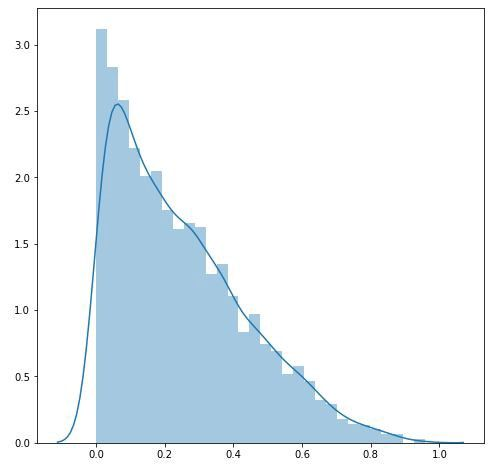An example of a Beta distribution graph. Plotted with Seaborn. | Image: Andrew Plummer

We can use the Box-Cox transformation to turn the above into as close to a normal distribution as the Box-Cox transformation permits.

``````tdata = boxcox(data)
plt.figure(figsize = (8, 8))
sns.distplot(tdata)
plt.show()``````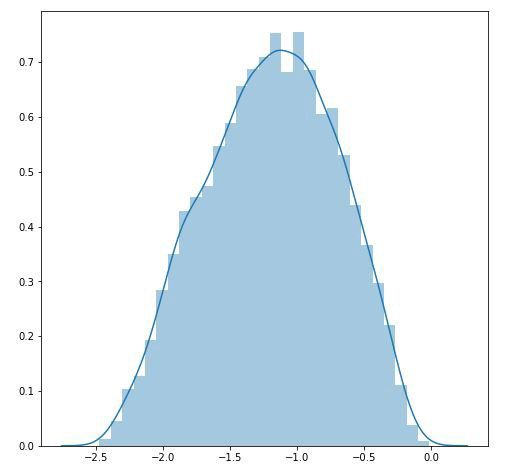A transformed normal distribution graph after applying Box-Cox transformation. Plotted with Seaborn. | Image: Andrew Plummer

And now our data looks more like a normal distribution.

## What Is the Box-Cox Transformation Equation?

If w is our transformed variable and “y” is our target variable, then the Box-Cox transformation equation looks like this: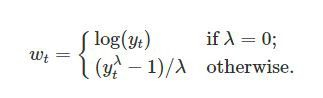Box-Cox transformation equation from Rob Hyndman’s and George Athanasopoulos’s book “Forecasting”. | Image: Andrew Plummer

In this equation, “t” is the time period and lambda is the parameter that we choose. You can also perform the Box-Cox transformation on non-time series data.

Notice what happens when lambda equals one. In that case, our data shifts down, but the shape of the data does not change. If the optimal value for lambda is one, then the data is already normally distributed, and the Box-Cox transformation is unnecessary.

More on DataWhat Is Descriptive Statistics?

### How Do You Choose Lambda?

We choose the value of lambda that provides the best approximation for the normal distribution of our response variable.

SciPy has a `boxcox` function that will choose the optimal value of lambda for us.

`scipy.stats.boxcox()`

Simply pass a 1-D array into the function and it will return the Box-Cox transformed array and the optimal value for lambda. You can also specify a number, alpha, which calculates the confidence interval for that value. For example, alpha = 0.05 gives the 95 percent confidence interval.

If llf is the log-likelihood function, then the confidence interval for lambda can be written as: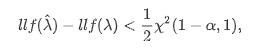From SciPy documentation on Box-Cox function. | Image: Andrew Plummer

In this equation, “X²” is the chi-squared distribution. It may also be unnecessary to transform your data if the confidence interval includes one.

Next, fit your model to the Box-Cox transformed data. You must revert your data to its original scale when you are ready to make predictions.

For example, your model might predict that the Box-Cox transformed value, given other features, is 1.5. You need to take that 1.5 and revert it to its original scale — the scale of your target variable.

Thankfully, SciPy also has a function for this.

``scipy.special.inv_boxcox(y, lambda)``

Enter the data you want to transform, “y,” and the lambda with which you had transformed your data.

[embedded content]

## Limits of Box-Cox Transformation

If interpretation is your goal, then the Box-Cox transformation may be a poor choice. If lambda is a non-zero number, then the transformed target variable may be more difficult to interpret than if we simply applied a log transform.

A second issue is that the Box-Cox transformation usually gives the median of the forecast distribution when we revert the transformed data to its original scale. Occasionally, we want the mean, not the median, and there are other ways to do that.

Now you know about the Box-Cox transformation, its implementation in Python, as well as its limitations.